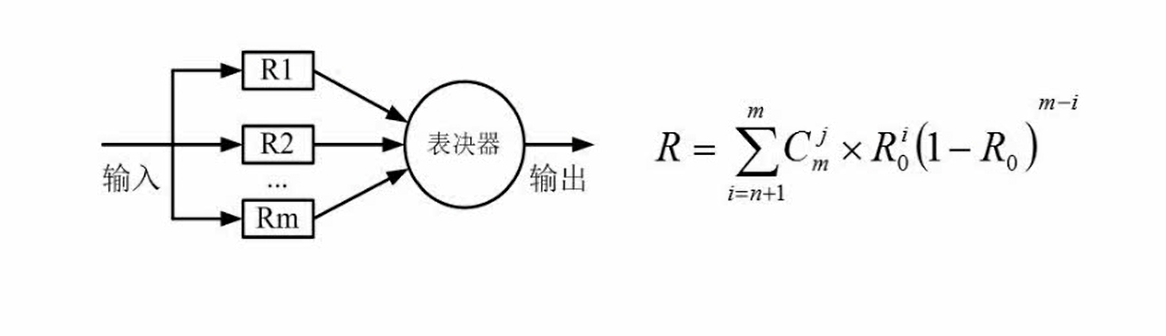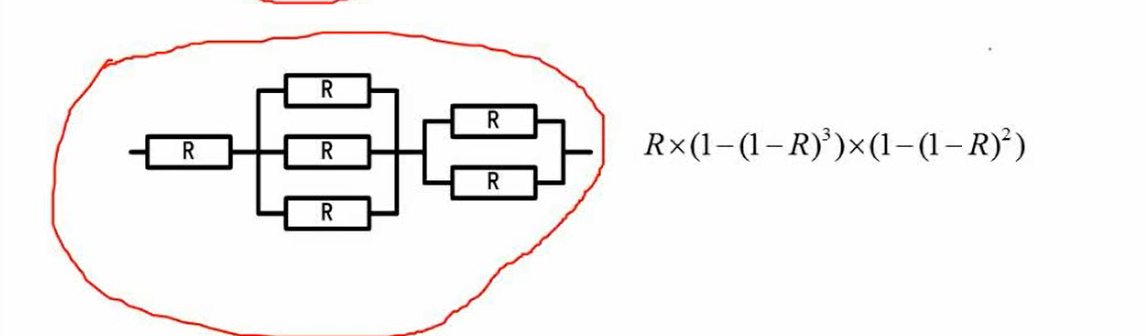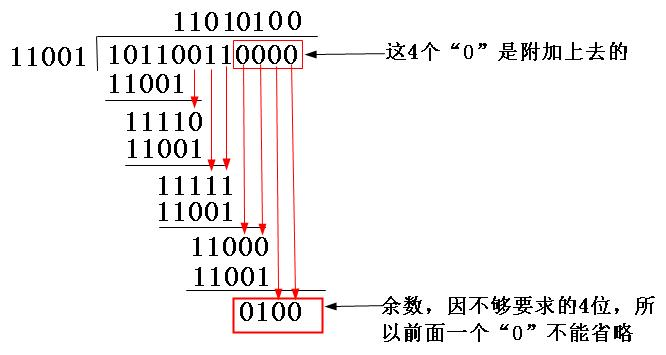# 数据的表示

## 不同进制的转换

• 其他进制转十进制，使用按权展开法
\begin{align}
4F(十六进制)=4 \times 16^1+16\times16^0=4\times16+16 =64+16 =80
\end{align}
• 十进制转其他进制，使用短除法
• 其他进制转其他进制
• 二进制转八进制，每三位划分开，然后将每三位都转成八进制
（二进制转十进制）
\begin{align}
101101B=&1\times2^0+0\times2^1+1\times2^2+1\times2^3+0\times2^4+1\times2^5 \\
=&1\times0+0\times2+1\times4+1\times8+0\times16+1\times32 \\
=&1+0+4+8+32 \\
=&45 \\
\end{align}
（二进制转八进制）
\begin{align}
101101B=&55O \\
\end{align}
• 二进制转十六进制，每四位划分开，然后将每四位都转成十六进制
（二进制转十六进制）
\begin{align}
101101B=&2DH \\
\end{align}

# 计算机结构

## CPU

### 运算器

• 算数逻辑单元ALU
• 累加寄存器AC（通用寄存器）
• 数据缓冲寄存器DR
• 状态条件寄存器PSW（用来存储运算过程中相关标记位）

### 控制器

• 程序计数器PC（了解下一条程序指令的位置）
• 指令寄存器IR
• 指令译码器
• 时序部件

## 存储器

### 高速缓存（Cache）

\begin{aligned}
&t_3=h_1\times{t_1}+(1-h)\times{t_2}\\
&其中，t_3代表使用”Cache+主存储器”的系统的平均周期，\\
&t_1代表Cache周期时间，即读写Cache中数据的时间\\
&t_2代表主存周期时间，即读取储存中数据的时间\\
&h代表访问Cache的命中率，即读取Cache时能够访问到数据的概率\\
\end{aligned}

• 磁盘存储器

• 光盘存储器

## 主存

• DRAM
• SRAM

• MROM
• PROM
• EPROM
• 闪速存储器

### 编址

84位的存储器
8
8位的存储器

（1） A.96 B.112 C.132 D.156
（2） A.4 B.8 C.16 D.24

\begin{aligned}
C7FFFH-AC000H+1&=C8000H-AC000H=1C000H\\
&=12\times16^3+1\times16^4\\
&=12\times4096+65536\\
&=114688(地址单元)\\
&=\frac{114688}{1024}\\
&=112(K个地址单元)
\end{aligned}

\begin{aligned}
K&=\frac{112K\times{16}}{28\times{16K}}\\
&=\frac{112\times{16}}{28\times16}\\
&=\frac{112}{28}\\
&=4
\end{aligned}

bit=bit,存储的bit=内存数据bit

# 计算机体系结构分类

## 从微观角度

————按并行程度进行划分————

SISD

SIMD

MISD

MIMD

• 字串行/字并行
• 位串行/位并行

# 指令系统

## 指令集体系结构的分类

• 操作数在CPU中的存储方式
即操作数从主存中取出后保存在什么地方
• 显示操作数的数量
即在典型的指令中有多少个显示命名的操作数。
• 操作数的位置
即任一个ALU指令的操作数是否能放在主存中，以及如何定位。
• 指令的操作
即在指令集中提供哪些操作。
• 操作数的类型与大小

## CISC（复杂指令集计算机）

• 指令集过于庞杂
• 处理速度降低
微程序技术是CISC的重要蜘蛛，每条复杂指令要通过执行一段解释性微程序才能完成，因此需要多个CPU周期，降低了处理速度。
• 编译程序冗长、复杂
由于指令系统过于庞大，使高级语言编译程序选择目标指令的范围过大，并使编译程序本身冗长、复杂，从而难以优化哈编译使之生成真正高效的目标代码。
• 动作繁杂、设计复杂、研制周期长
CISC强调完善的中断控制，势必导致动作反动、设计复杂、研制周期长
• 出错几率增大
CISC给芯片设计带来很多困难，使芯片种类增多，出错几率增大，成本提高而成本率降低。

CISC是早期的设计方案，为的是为不同的用户进行定制，因此可以实现不同的用户不同的指令。

## RISC（精简指令集计算机）

• 重叠寄存器窗口技术
• 优化编译技术
• 超流水及超标量技术
• 在微程序技术中结合硬布线逻辑与微程序

## 流水线技术

• 顺序方式
• 重叠方式： 通常一次重叠
• 流水方式

### 流水线计算

• 未使用流水线

 取指令 1 2 3 4 分析指令 1 2 3 4 执行指令 1 2 3 4
• 使用流水线执行指令

 取指令 1 2 3 4 5 6 7 8 9 10 分析指令 1 2 3 4 5 6 7 8 9 10 执行指令 1 2 3 4 5 6 7 8 9 10
1. 流水线周期$(\Delta t)$为执行周期最长的一个执行阶段
2. 流水线计算公式为：1条指令执行时间+（指令条数-1）*流水线周期

• 理论公式：$T=(t_1+t_2+…+t_k)+(n-1)\times \Delta t$
$k代表的是执行指令经历的阶段数量，t_1,t_2…,t_n代表每一个阶段所花费的时间，n代表执行指令的条数$

• 实际公式：$T=(k+n-1)\times \Delta t$
其中$\Delta t为流水线周期，也就是执行周期里最长的一个阶段$

例：若指令流水线把一条指令分为取指、分析和执行三部分，且三部分的时间分别是取值2ns，分析2ns，执行1ns。那么流水线周期是多少？100条指令全部执行完毕需要的时间是多少？

\begin{aligned}
T=(2+2+1)+(100-1)\times{2}=5+99\times{2}=5+198=203(ns)
\end{aligned}

\begin{aligned}
T=(3+100-1)\times{2}=102\times{2}=204(ns)
\end{aligned}

\begin{aligned}
T=(2+2+1)\times{100}=500(ns)
\end{aligned}

### 流水线吞吐率计算

\begin{align} T_p= \frac{指令条数}{流水线执行时间} \end{align}

\begin{aligned}
T_p=100\times{203}
\end{aligned}

### 流水线最大吞吐率计算

\begin{align} TP_{max}=\lim\limits_{N\to\infty}\frac{n}{(k+n-1)\times\Delta{t}}\approx\frac{1}{\Delta{t}} \end{align}

\begin{aligned}
TP_{max}&=\lim\limits_{N\to\infty}\frac{100}{3+100-1\times{2}}\\
&=\lim\limits_{N\to\infty}\frac{100}{102\times{2}}\\
&=\lim\limits_{N\to\infty}\frac{100}{204}\\
&\approx{\frac{1}{2}}
\end{aligned}

### 流水线加速比

\begin{align} S=\frac{不使用流水线的执行时间}{使用流水线的执行时间} \end{align}

\begin{aligned}
S=\frac{500}{203}\approx2.5
\end{aligned}

### 流水线效率

\begin{align} E=\frac{N个任务占用的时空区}{K个流水段的总时空区}=\frac{T_0}{kT_k} \end{align}

\begin{aligned}
E=\frac{3\times{10}-2\times{3}}{3\times{10}}=\frac{24}{30}=0.8
\end{aligned}

# 可靠性

## 串联系统的可靠度计算

\begin{aligned}
&{输入}\rightarrow\boxed{R_1}\rightarrow \boxed{R_2} \rightarrow \boxed{R_3} … \rightarrow \boxed{R_n} \rightarrow 输出 \\
&R=R_1\times{R_2}\times{R_3}\times{…}\times{R_n} \\
&\lambda={\lambda}_1+{\lambda}_2+…+{\lambda}_n \\
\end{aligned}
R为总的串联系统可靠度，R_x为每个系统可靠度,$\lambda为总的失效率，而\lambda_x为每个系统的失效率，即单位时间内失效的元件数与元件总数的比例$

## 并联系统的可靠度计算

\begin{aligned}
&R=1-(1-R_1)\times{1-R_2}\times{…}\times(1-R_n) \\
&\mu=\frac{1}{\frac{1}{\lambda}\sum\limits_{j=1}^{n}{\frac{1}{j}}} \\
\end{aligned}

## 模冗余系统m为子系统的个数，i为相应的值

## 混合系统# 差错控制

## 码距

1、在一个码组内为了检测e个误码，要求最小码距d应该满足，d>=e+1
2、在一个码组内为了纠正t个误码，要求最小码距d应该满足，d>=2t+1

## 奇偶校验码

1 11100000
1 11000000

1、只能检测出奇数位错误，无法检测出偶数位错误
2、只能检测出错误，无法检测出错误的地方

## CRC

0~r 0~k
n n-1 …r+1 r r-1 … 1

CRC的校验步骤有：

1、先选择一个多项式，用于在接收端进行校验时对数据帧进行校验。
2、在要发送的数据帧后面加k-1个0，（k为选定的多项式位数），并用加完之后的数据帧以“模二除法”方式除以多项式，余数便是该帧的CRC校验码。
3、把校验码加在原数据位上，即生成了最后的数据帧，把这个新的帧发到了接收端，接收端对这个新帧以“模二除法”除以之前选择的除数，如果没有余数，则表明该帧在传输过程中没有出错，否则便出了差错。
T mod P == 0 ……(1)

### 模二除法

CRC可以检错，但不可以纠错## 海明校验码

将海明码下标转换为二进制，则可有n+k个长度为k的数，那么
P(i)=H(1)+H(2)+H(3) (这里是所有第i位为1的值进行异或操作)

### 校验海明码的步骤

1. 在数据位间插入k个校验位，k的值符合$2^k-1>=n+k$（n为数据位的位数，k位校验位的位数），则n+k为海明码位数。
2. 确定插入位置，即每一个校验位在海明码中的位置，假设每一位的位置为P(i)（每一位的值为P(i)）,而他在海明码中的位置为H(j)，则$j=2^{i-1}$
3、确定每一个校验位的值，将每一位H(j)化为1+2+4+..2^n的形式，即1+2+4+..2^n=j，然后对每一个

## 计算机安全

1. 机密性
2. 完整性
3. 可用性
4. 可控性
5. 可审查性

### 计算机加密技术

#### 对称加密算法

• 数据加密标准(Data Encryption Standard) 主要采用替换和移位的方法加密
• 三重DES 相当于将密钥的长度加倍。
• 国际数据加密算法(IDEA)
• 高级加密标准(AES) 基于排列和置换运算。排列是对数据重新进行安排，置换是将一个数据单元替换为另一个数据单元。

RSA算法

### 计算机认证技术

#### 关键绩效指标

• 认证机构：即数字证书的申请及签发机关
• 数字证书库：存储已签发的数字证书及公钥
• 密钥备份及恢复系统
• 证书作废系统
• 应用接口